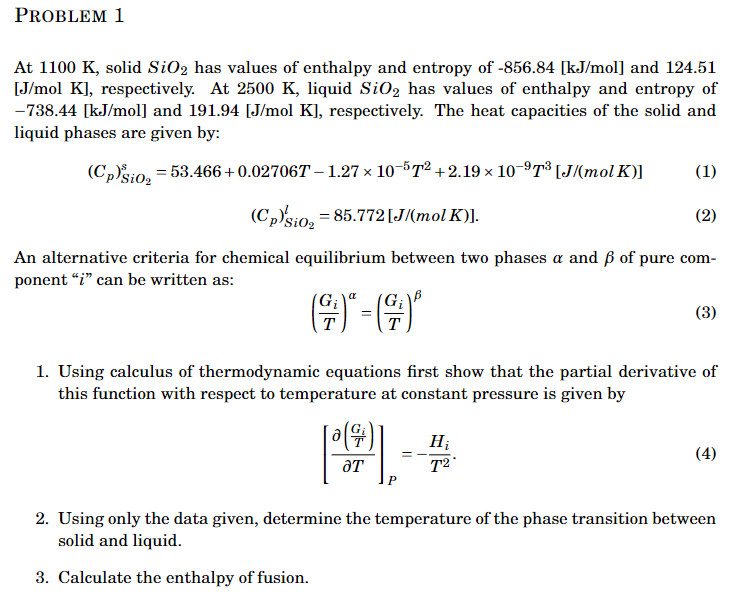Home / Expert Answers / Chemical Engineering / at-1100k-solid-sio2-has-values-of-enthalpy-and-entropy-of-856-84-kj-mol-and-124-51-j-pa692

# (Solved): At 1100K, solid SiO2 has values of enthalpy and entropy of 856.84[kJ/mol] and 124.51 [J/ ...At , solid has values of enthalpy and entropy of and 124.51 [J/mol K], respectively. At , liquid has values of enthalpy and entropy of and , respectively. The heat capacities of the solid and liquid phases are given by: An alternative criteria for chemical equilibrium between two phases and of pure component " " can be written as: 1. Using calculus of thermodynamic equations first show that the partial derivative of this function with respect to temperature at constant pressure is given by 2. Using only the data given, determine the temperature of the phase transition between solid and liquid. 3. Calculate the enthalpy of fusion.

We have an Answer from Expert

1) To solve this problem, we'll need to apply the calculus of thermodynamic equations and use the given data. Let's go step by step.
First, we need to differentiate the alternative criteria for chemical equilibrium with respect to temperature at constant pressure:

Applying the chain rule:

Simplifying:

Dividing both sides by (dG_i / dT|P):    ? = ?1 This implies that ? = ?1, which means the criterion for chemical equilibrium between the solid and liquid phases is satisfied when ? = ?1.
We have an Answer from Expert